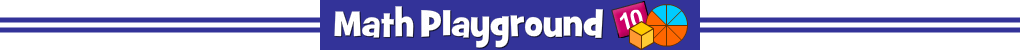Help the Galaxy Pals refuel their spaceships.
The total number of fuel rods is shown on the storage facility (bottom left).
Each spaceship shows its own number of fuel rods.
Aim the fuel blaster toward the spaceship that will make the given total.
Galaxy Pals Fraction Number Pairs - Learning Connections
Essential Skills
Mental Math - practice adding fraction numbers

Common Core Connection for Grade 4
Understand addition of fractions as joining parts referring to the same whole.
Understand two fractions as equivalent if they are the same size.
Recognize and generate simple equivalent fractions, e.g., 1/2 = 2/4, 4/6 = 2/3.
More Math Games to Play